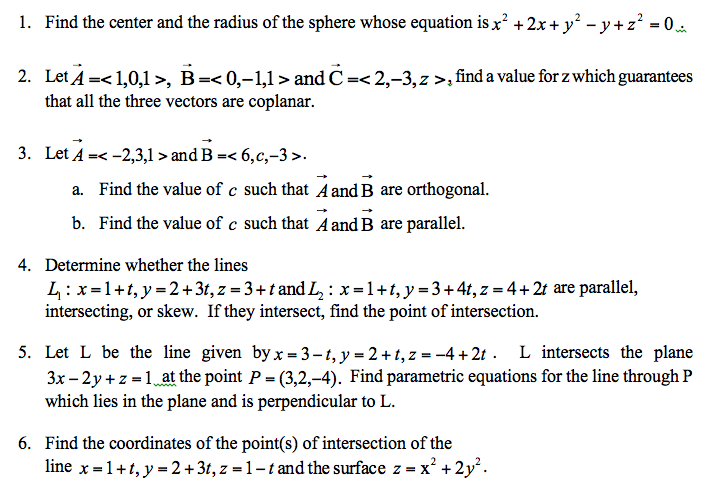1
0
watching
79
views10 Nov 2019

# This is for math 254. thanks for ur help!Find the center and the radius of the sphere whose equation is x2 + 2x + y2 - y + z2 = 0. Let A rightarrow =, B rightarrow = and C rightarrow =, find a value for z which guarantees that all the three vectors are coplanar. Let A rightarrow = and B rightarrow =. Find the value of c such that A rightarrow and B rightarrow are orthogonal. Find the value of c such that A rightarrow and B rightarrow are parallel. Determine whether the lines L1 : x = l + t,.y = 2 + 3t, z = 3 + t and L2 : x = l + t, y = 3 + 4t, z = 4 + 2t are parallel, intersecting, or skew. If they intersect, find the point of intersection. Let L be the line given by x = 3 - t, y = 2 + t, z = -4 + 2t. L intersects the plane 3x - 2y + z = 1 at the point P = (3,2,-4). Find parametric equations for the line through P which lies in the plane and is perpendicular to L. Find the coordinates of the point(s) of intersection of the line x = l + t, y = 2 + 3t, z = l - t and the surface z = x2 + 2y2.

For unlimited access to Homework Help, a Homework+ subscription is required.Jarrod RobelLv2
19 Apr 2019

## Unlock all answers

Get 1 free homework help answer.